•5星
163KB qq_41934573 2021-05-04 14:18:22
•5星
111.52MB qq_28239511 2021-03-17 13:04:53
•5星
12.18MB qq_44872173 2021-03-04 10:29:40
•5星
566KB ambiguous__ 2021-04-02 17:37:43
•5星
387KB weixin_44510615 2021-06-23 18:04:15
•5星
14.01MB qq_27595745 2021-08-08 21:31:06
•5星
29KB m0_52957036 2020-04-29 07:50:59
•5星
47KB m0_52957036 2020-04-29 07:50:55
•5星
114B u012225745 2021-07-24 10:47:05
•5星
203.91MB weixin_37932346 2021-04-24 01:17:07
• 主成分分析 1，它提供的是一个或者几个综合指标 指标要求：线性组合，信息不重合（协方差和相关系数为0），按重要性排序（重要性由方差来刻画） 2，这些综合指标是由原来的变量通过线性组合/加权平均构成的 3，它...

主成分分析

1，它提供的是一个或者几个综合指标

• 指标要求：线性组合，信息不重合（协方差和相关系数为0），按重要性排序（重要性由方差来刻画）

2，这些综合指标是由原来的变量通过线性组合/加权平均构成的

3，它的目的是最大成分的区分你这个群体当中的最大的个体

一，怎么找出指标

Y1     Y2      Y3       Y4        Y5

Z1=（a1Y1+a2Y2+a3Y3+........）

Z2

Z2

目标

（1）最大化方差，按照有大到小排序

（2）z1 z2互不相关

保证  Z 的方差最大，协方差为0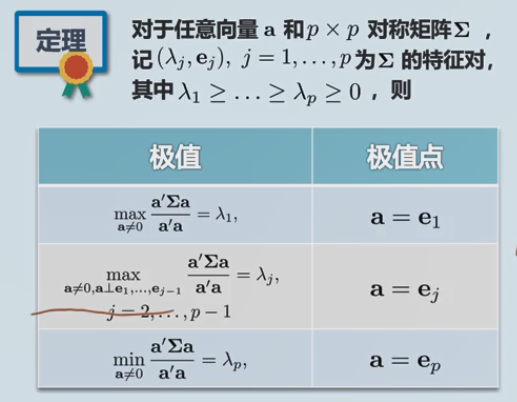取到的主成分对应的信息含量就是他的特征值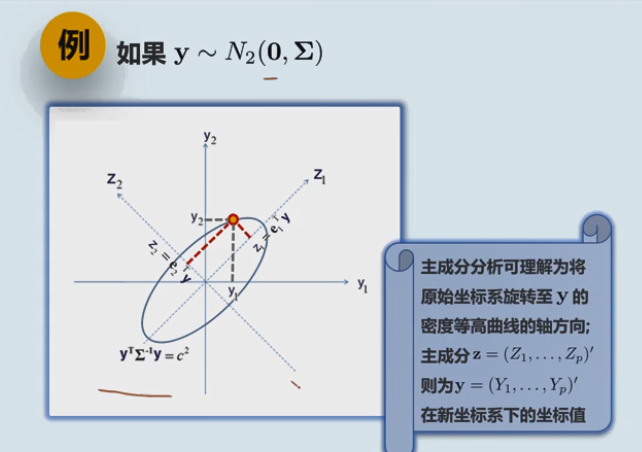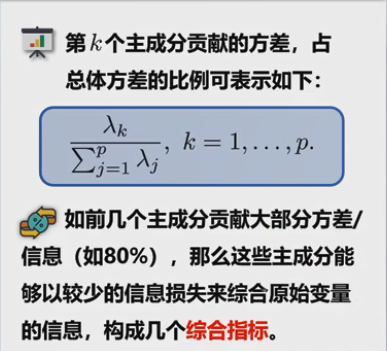展开全文XU_MAN_ 2020-03-11 15:09:48
• 216KB dp2016 2019-09-24 23:27:47
• 花了好几天的时间去学习数据挖掘里面的主成分分析（principal component analysis , PCA）。PCA是一种常用的无监督学习方法，他作为一种数据降维的方法是很有效的。选择的数据集很经常的都是高维数据，处理起来要么...

1.前言
花了好几天的时间去学习数据挖掘里面的主成分分析（principal component analysis , PCA）。PCA是一种常用的无监督学习方法，他作为一种数据降维的方法是很有效的。选择的数据集很经常的都是高维数据，处理起来要么就是处理时间太慢，要么就是训练出来的学习模型精度会很低。同时，这些高维数据里面就有的数据是不相关的，这些特征（维）是我们不需要的。那么对于这种情况，降维是必须的。

2.主成分分析算法
据《统计学习方法》一书的表达：“主成分分析是利用正交变换把由线性相关变量表示的观测数据转换为少数几个由线性无关变量表示的数据，线性无关的变量称为主成分。”按我的理解，就是将所有的高维数据点，通过投影映射的方法，将数据点转换至一个新的坐标系，而这个坐标系的维度是小于或等于原先的坐标系维度的。同时，找到这个我们想要的坐标系的标准就是，在新坐标系中，投影后的数据对应的每个坐标轴上的数据的方差和都是最大的，这样我们保存下来的数据就是最完整的。而为了找到这个坐标轴，我们可以拆分子问题，先找第一个坐标轴，第二个…知道找到想要的结果。这里的第一坐标轴，就称为是第一主成分，以此类推。

3.总体主成分的定义(a = (a1 , a2 , … , ak)T ，其中a1 , a2 , … 都是一组n维向量)T：
满足以下三点：
一、每一个向量a1都是单位向量
二、a矩阵中的每一个向量a1 ， a2 ， …两两的协方差均为0，即他们之间互不相关
三、a1是x数据的所有线性变换中方差最大的，a2是与a1无关的x的所有线性变换中方差和最大的…

4.数学推导
一、针对某个均值为0的数据集x中，找到一个相对于当前x方差最大的主成分，也就是一个坐标轴，坐标轴的方向向量是w = (w1 , w2 , w3 ,…,wn)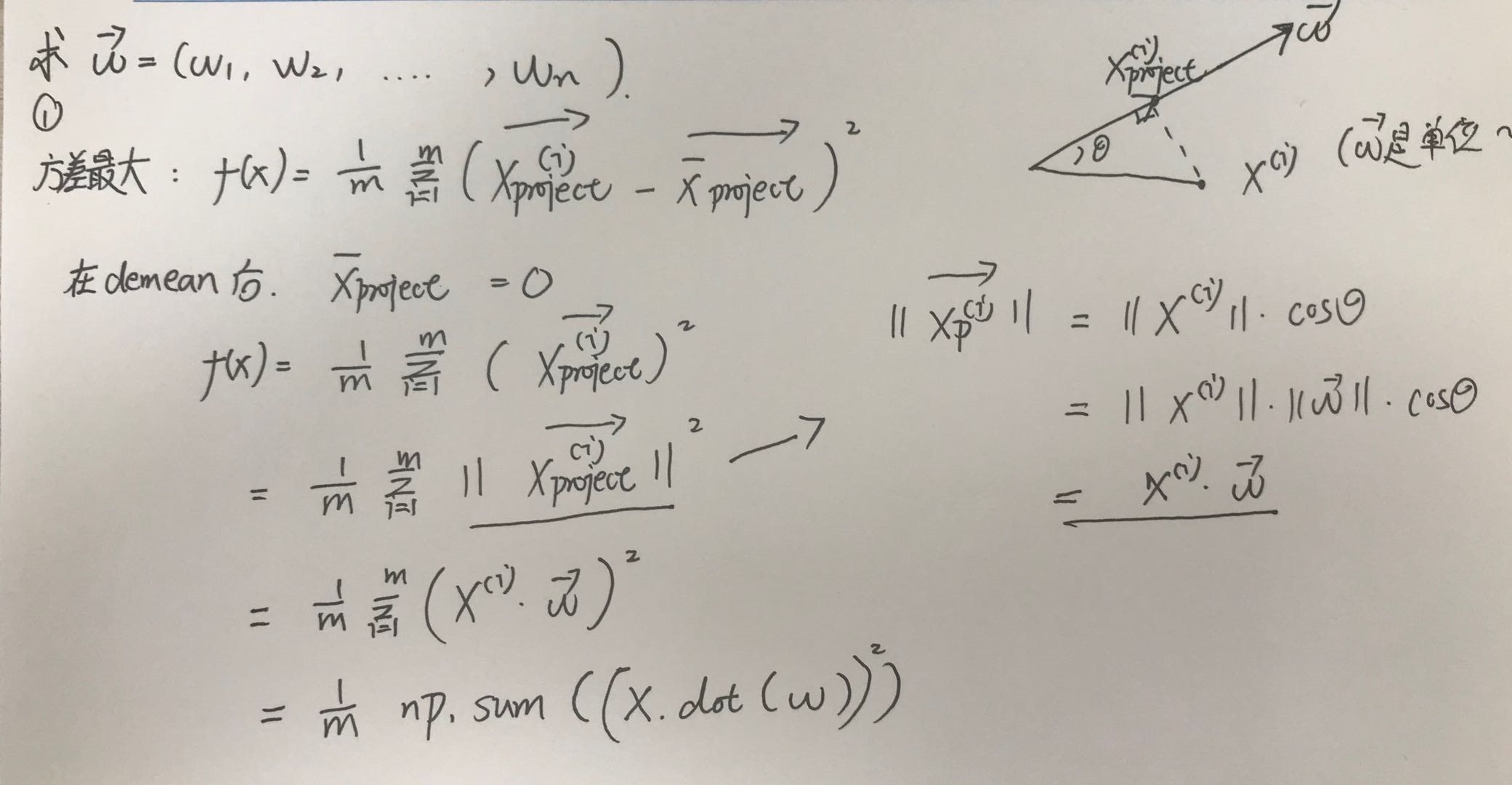得到方差函数f(x)
二、针对当前的方差函数，求出他的最大值，即方差最大化，用的是梯度上升的方法进行寻找。对多元函数的极值，还是同样的方法，求偏导。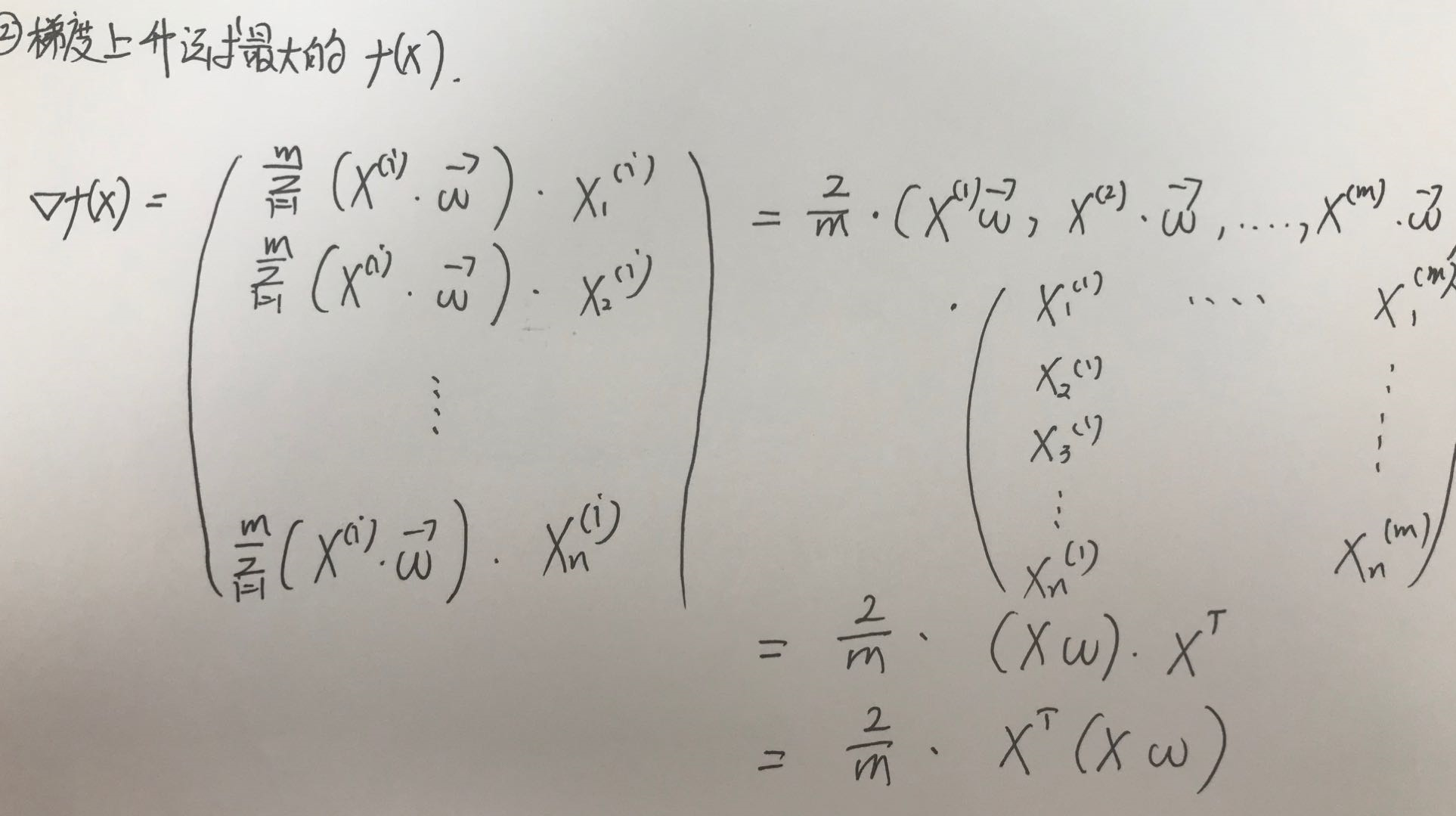三、当求出一个主成分，想求下一个主成分，此时应先对当前的x进行操作，就是使得新的x与刚刚的主成分无关，这样才确保求出来的新的主成分是和原来的主成分是不相关的。

四、不断循环上述过程，直到得到自己预想数量的总体主成分

5.PCA代码

import numpy as np

class PCA:
def __init__(self , n_components):
assert n_components >= 1 , "The number of n is not valid."
self.n_components = n_components
self.components_ = None

#对这个PCA实例，传入一个待主成分分析的矩阵X，用PCA来适应这个X，提取出他的k个主成分（k个方向向量）
#保存再PCA实例的components里面，是个k*n维矩阵，k是主成分的个数，n是X的列数
def fit_(self , X1):

assert X1.shape >= self.n_components , "It's not legal."

# 对数据进行demean操作，使得数据在各个特征下的均值为0
def demean(X):
return X - np.mean(X , axis = 0)

# 求数据点投影到一个方向向量后的方差，这个函数越大越可取
def f(X , w):
return ((np.sum(X.dot(w))) ** 2 ) / X.shape

# 求方差的导数，用这个得到一个对w向量每一个项的导数，返回的也是一个n维的向量
def df(X , w):
return (X.T.dot(X.dot(w))) * 2. / X.shape

# 对w进行单位化
def direction(w):
return w / np.linalg.norm(w)

# 得到下一个主成分的方法，就是将原先的数据点除去前一个主成分方向上的数值，具体就是
# 针对某一个点X(i)，他在前一个主成分W上的投影是Xproject，然后紧接着就可以做差X(i) - X(i)project得到去除第一主成分的矩阵
def delete_pca(X , w):
X2 = np.empty(X.shape)
for i in range(0 , X.shape):
X2[i] = X[i] - (X[i].dot(w)) * w
return X2

##梯度上升法求出最佳的向量w，也就是最佳的参数
def get_next_pca(X , initial_w , eta = 0.01 , n_iter = 1e4 , epional = 1e-8):

w = direction(initial_w)
count = 0
while count < n_iter:
history_w = w
w = w + eta * gradient
w = direction(w)
if(abs(f(X , history_w) - f(X , w)) < epional):
break
count += 1
return w

# 如果是想得到n个主成分，通过一次调用函数就想得出
def get_n_pca(X, n , eta = 0.01 , n_iter = 1e4 , epional = 1e-8):
X_pca = X.copy()

#注意这里的W矩阵（里面包括n个方向向量w，即前n个主成分）的矩阵大小，一共有n行（前n个主成分），并且有X.shape行
total_w = np.empty((self.n_components , X.shape))
for i in range(0 , n):
initial_w = np.random.random(X.shape)
w = get_next_pca(X_pca , initial_w)
for j in range(0 , len(w)):
total_w[i,j] = w[j]
X_pca = delete_pca(X_pca , w)

X1 = demean(X1)
self.components_ = get_n_pca(X1 , self.n_components)

return self

#当PCA已经fit之后，可以用此PCA来转化X得到将维后的矩阵X
def tranport(self , X):
print(self.components_)
return X.dot(self.components_.T)

6.scikit-learn封装的PCA类使用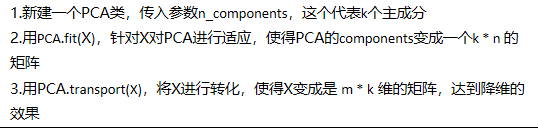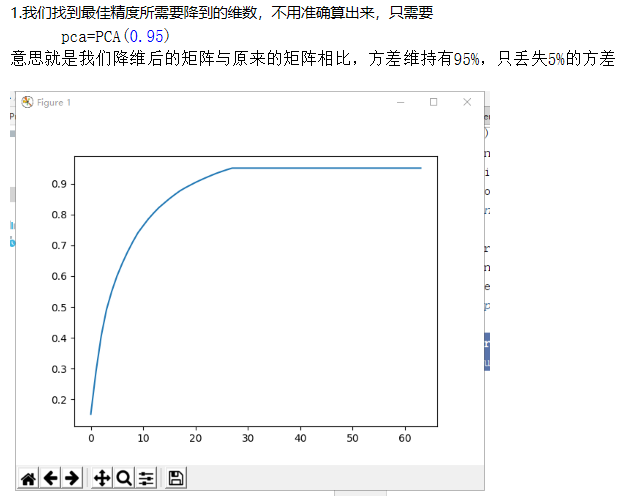pca = PCA(0.95)
pca.fit(X_train)
X_train_reduction = pca.transform(X_train)
X_test_reduction = pca.transform(X_test)

展开全文m0_37860003 2019-07-27 16:24:27
• 参考：https://blog.csdn.net/lswbjtu/article/details/72764141 ... http://muchong.com/t-9070350-1 一、理论 （一）主成分分析的作用 （二）准备工作：数据标准化 （...

http://www.360doc.com/content/14/1103/09/17553313_422099670.shtml

http://muchong.com/t-9070350-1

一、理论

（一）主成分分析的作用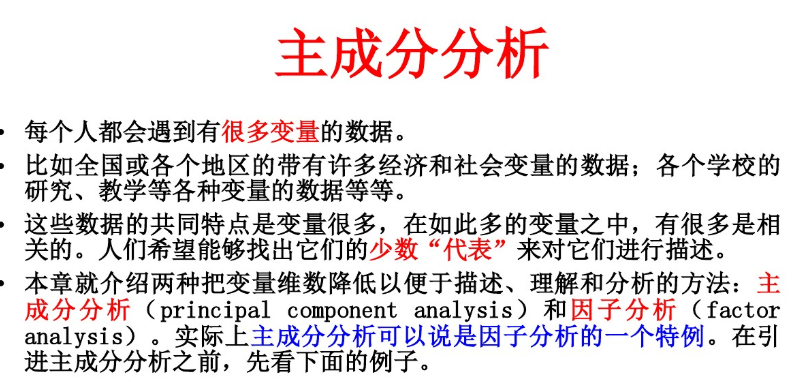（二）准备工作：数据标准化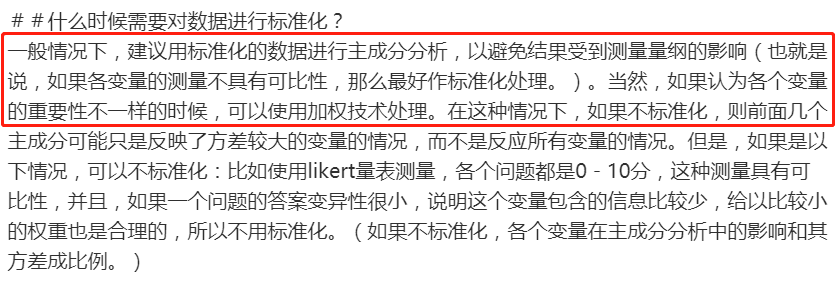（三）实验（R）

apply(USArrests,2,mean)
##   Murder  Assault UrbanPop     Rape
##    7.788  170.760   65.540   21.232
apply(USArrests,2,var)
##     Murder    Assault   UrbanPop       Rape
##   18.97047 6945.16571  209.51878   87.72916
##发现均值和方差的差异较大，因此必须进行标准化

scale(USArrests,center = T,scale = T)
##然后进行PCA分析，标准化操作：scale=T
pr.out<-prcomp(USArrests,scale=T)
names(pr.out)
##  "sdev"     "rotation" "center"   "scale"    "x"
##center和scale是标准化前的均值和标准差
##rotation包含了主成分载荷信息，列向量是主成分载荷向量

pr.out$rotation ## PC1 PC2 PC3 PC4 ## Murder -0.5358995 0.4181809 -0.3412327 0.64922780 ## Assault -0.5831836 0.1879856 -0.2681484 -0.74340748 ## UrbanPop -0.2781909 -0.8728062 -0.3780158 0.13387773 ## Rape -0.5434321 -0.1673186 0.8177779 0.08902432 ##主成分负荷系数(principal component loadings)表示各个成分和原来变量之间的相关程度 biplot(pr.out,scale=0) ##前两个主成分的双标图 得前两个主成分的双标图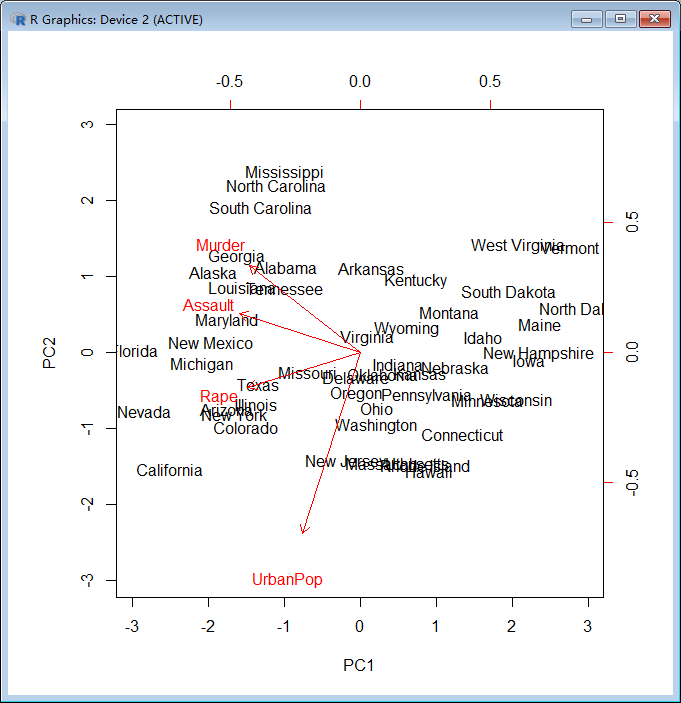biplot双标图，这个图可以把成分负荷、因子得分都反应在一个图上面。因为是平面图，因此只能指定两个成分，比如想呈现1、2成分，可以使用:>biplot(fit2.pca,c(2,4))。图中两个坐标对应各自的成分，红色的箭头的长度表示负荷的长度，方向表示符合的符号是正还是负，而各个点是各个个案对应的成分得分。（详细的说明，可以到splus的主页现在说明文档）。点之间的距离，反映它们对应的样本之间的差异大小，两点相距较远，对应样本差异大；两点相距较近，对应样本差异小，存在相似性。 2、每个主成分的标准差、每个主成分的方差解释比例、绘制每个主成分的PVE和累积PVE图 ##主成分的标准差 pr.out$sdev ##一般来说，第一主成分的方差>第二主成分的方差>.....
##  1.5748783 0.9948694 0.5971291 0.4164494
pr.out$var<-pr.out$sdev^2

##计算每个主成分的方差解释比例
pve<-pr.out$var/sum(pr.out$var)
pve

##  0.62006039 0.24744129 0.08914080 0.04335752
##绘制每个主成分的PVE和累积PVE图
par(mfrow=c(1,2))
plot(pve,xlab = "Principal Component",ylab="Proportion of Variance Explained",
ylim=c(0,1),type='b')
plot(cumsum(pve),xlab = "Principal Component",ylab="Proportion of Variance Explained",
ylim=c(0,1),type='b')

主成分对于总方差的贡献比例(Proportion of Variance Explained,简记 PVE)

PVE图和累积PVE图可以用于选择主成分，一般选取前几个能解释大部分数据方差的主成分。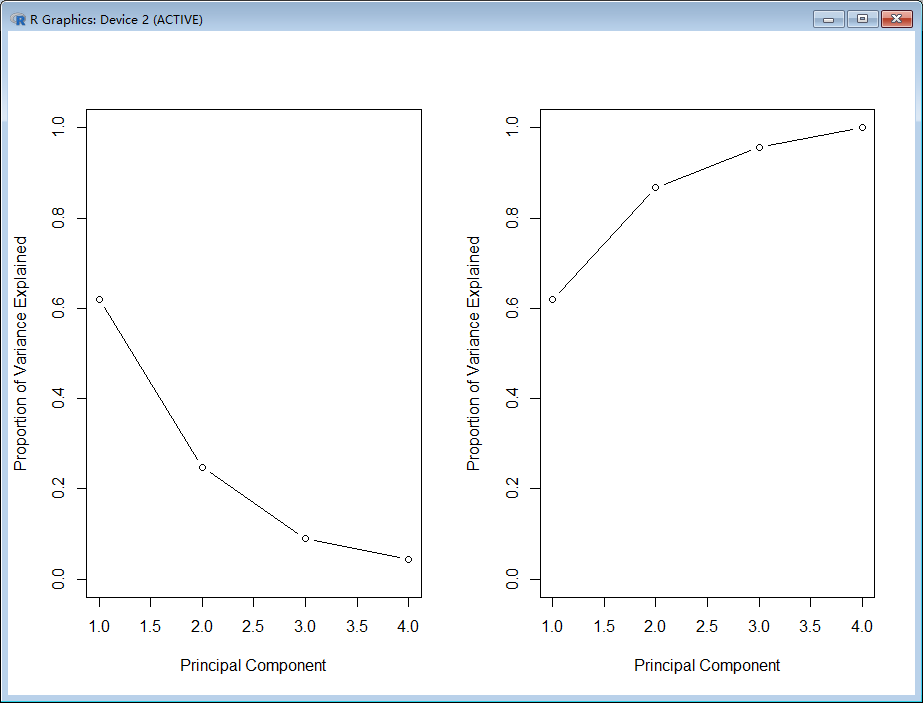展开全文YWP_2016 2020-03-25 19:25:28
• 数据挖掘—主成分分析法降维和最小最大规范化 算法 java

1)将原始数据按列组成n行m列矩阵X 2)特征中心化。即每一维的数据都减去该维的均值，使每一维的均值都为0 3)求出协方差矩阵 4)求出协方差矩阵的特征值及对应的特征向量 5)将特征向量按对应的特征值大小从上往下按行...
• 算法步骤:
• 1)将原始数据按列组成n行m列矩阵X
• 2)特征中心化。即每一维的数据都减去该维的均值，使每一维的均值都为0
• 3)求出协方差矩阵
• 4)求出协方差矩阵的特征值及对应的特征向量
• 5)将特征向量按对应的特征值大小从上往下按行排列成矩阵，取前k行组成矩阵p
• 6)Y=PX 即为降维到k维后的数据

PCA

/*
* 算法步骤:
* 1)将原始数据按列组成n行m列矩阵X
* 2)特征中心化。即每一维的数据都减去该维的均值，使每一维的均值都为0
* 3)求出协方差矩阵
* 4)求出协方差矩阵的特征值及对应的特征向量
* 5)将特征向量按对应的特征值大小从上往下按行排列成矩阵，取前k行组成矩阵p
* 6)Y=PX 即为降维到k维后的数据
*/
public class PCA {
public static DenseMatrix64F runPCA(DenseMatrix64F src,int k) {

DenseMatrix64F rs = new DenseMatrix64F(src.numRows,k);

//计算输入矩阵每个元素和特征值平均的差值矩阵
DenseMatrix64F norm_X = new DenseMatrix64F(src.numRows,src.numCols);

for(int i =0;i<src.numCols;i++) {
double tmp=0;
for(int j=0;j<src.numRows;j++) {
tmp+=src.get(j, i);
}
tmp /=src.numRows;
for(int j=0;j<src.numRows;j++) {
norm_X.set(j,i, src.get(j, i)-tmp);
}
}

//计算协方差矩阵
DenseMatrix64F norm_X_T = new DenseMatrix64F(src.numCols,src.numRows);

CommonOps.transpose(norm_X, norm_X_T);

DenseMatrix64F scatter_matrix = new DenseMatrix64F(src.numCols,src.numCols);

CommonOps.mult(norm_X_T,norm_X,scatter_matrix);

//特征向量分解
EDInfo ed = JacobiCount(new DenseMatrix64F(scatter_matrix),0.001,1000);
//选取前k个特征
DenseMatrix64F feature = new DenseMatrix64F(k,src.numCols);

for(int i=0;i<k;i++) {
for(int j=0;j<src.numCols;j++) {
feature.set(i, j, ed.getValues().get(j, i));
}
}

DenseMatrix64F feature_T = new DenseMatrix64F(src.numCols,k);

CommonOps.transpose(feature, feature_T);

CommonOps.mult(norm_X,feature_T,rs);

return rs;
}
public static EDInfo JacobiCount(DenseMatrix64F src, double diff, int iter) {

DenseMatrix64F values = new DenseMatrix64F(src.numRows,src.numCols);

for(int i=0;i<src.numRows;i++) {
for(int j=0;j<src.numCols;j++) {
if(i == j) {
values.set(i, j, 1);
}else {
values.set(i, j, 0);
}
}
}

int nCount = 0;

while(true)
{

double dbMax = Double.MIN_VALUE;
int nRow = 0;
int nCol = 1;

for(int i=0;i<src.numRows;i++) {
for(int j=0;j<src.numCols;j++) {
if(i != j && Math.abs(src.get(i, j)) > dbMax) {
dbMax = Math.abs(src.get(i, j));
nRow = i;
nCol = j;
}
}
}

if(dbMax < diff)
break;

if(nCount > iter)
break;

nCount++;

double dbApp = src.get(nRow, nRow);
double dbApq = src.get(nRow, nCol);
double dbAqq = src.get(nCol, nCol);

double dbAngle = 0.5*Math.atan2(-2*dbApq,dbAqq-dbApp);
double dbSinTheta = Math.sin(dbAngle);
double dbCosTheta = Math.cos(dbAngle);
double dbSin2Theta = Math.sin(2*dbAngle);
double dbCos2Theta = Math.cos(2*dbAngle);

src.set(nRow, nRow, dbApp*dbCosTheta*dbCosTheta +
dbAqq*dbSinTheta*dbSinTheta + 2*dbApq*dbCosTheta*dbSinTheta);
src.set(nCol, nCol, dbApp*dbSinTheta*dbSinTheta +
dbAqq*dbCosTheta*dbCosTheta - 2*dbApq*dbCosTheta*dbSinTheta);
src.set(nRow, nCol, 0.5*(dbAqq-dbApp)*dbSin2Theta + dbApq*dbCos2Theta);
src.set(nCol, nRow,src.get(nRow, nCol));

for(int i = 0; i < src.numRows; i ++)
{
if((i!=nCol) && (i!=nRow))
{
dbMax = src.get(i, nRow);
src.set(i, nRow,src.get(i, nCol)*dbSinTheta+dbMax*dbCosTheta);
src.set(i, nCol,src.get(i, nCol)*dbCosTheta-dbMax*dbSinTheta);
}
}

for (int j = 0; j < src.numRows; j ++)
{
if((j!=nCol) && (j!=nRow))
{
dbMax = src.get(nRow, j);
src.set(nRow, j,src.get(nCol, j)*dbSinTheta+dbMax*dbCosTheta);
src.set(nCol, j,src.get(nCol, j)*dbCosTheta-dbMax*dbSinTheta);
}
}

for(int i = 0; i < src.numRows; i ++)
{
dbMax = values.get(i,nRow);
values.set(i, nRow,values.get(i,nCol)*dbSinTheta+dbMax*dbCosTheta);
values.set(i,nCol,values.get(i,nCol)*dbCosTheta-dbMax*dbSinTheta);
}

}

double[] eig = new double[src.numRows];

for(int i=0;i<src.numRows;i++) {
eig[i] = src.get(i, i);
}

int[] sortInx = argsort(eig);

DenseMatrix64F tmpValues = new DenseMatrix64F(src.numRows,src.numCols);

for(int i=0;i<src.numRows;i++) {

for(int j=0;j<src.numRows;j++) {

tmpValues.set(i, j, values.get(j,sortInx[i]));
}

eig[i] = src.get(sortInx[i],sortInx[i]);

}

for(int i = 0; i < src.numRows; i ++)
{
double dSumVec = 0;
for(int j = 0; j < src.numRows; j ++)
dSumVec += tmpValues.get(j, i);
if(dSumVec<0)
{
for(int j = 0;j < src.numRows; j ++)
tmpValues.set(j, i,tmpValues.get(j, i)*-1);
}
}

return new EDInfo(tmpValues,eig);

}
public static int[] argsort(double[] input) {

int[] rs =  new int[input.length];

for(int i=0;i<input.length;i++){
rs[i] = i;
}

for(int i=0;i<input.length-1;i++) {
for(int j=i+1;j<input.length;j++) {
if(input[i] < input[j]) {

double tmp = input[i];
int tmpIndex = rs[j];

input[i] = input[j];
input[j] = tmp;

rs[j] = rs[i];
rs[i] = tmpIndex;

}
}
}

return rs;
}
static ArrayList<String> tempc=new ArrayList<>();
public  double[][] readData() throws IOException {
double[][] res=new double;
try { // 防止文件建立或读取失败，用catch捕捉错误并打印，也可以throw

/* 读入TXT文件 */

File filename = new File("src/bp/test.txt"); // 要读取以上路径的input。txt文件
String line = "";
int j=0;
while (line != null) {

String[] temp=line.split(",");
for(int i=0;i<13;i++)
{
res[j][i]=Double.parseDouble(temp[i]);
System.out.print( res[j][i]+" ");
}
System.out.println();
j++;
}

} catch (Exception e) {
e.printStackTrace();
}
return res;
}
public static void writeTxt( DenseMatrix64F denseMatrix64F){
try { // 防止文件建立或读取失败，用catch捕捉错误并打印，也可以throw

/* 读入TXT文件 */

StringBuilder stringBuilder=new StringBuilder();
for(int i=0;i<denseMatrix64F.numRows;i++)
{
for(int j=0;j<denseMatrix64F.numCols;j++)
stringBuilder.append(denseMatrix64F.get(i,j)).append(',');
stringBuilder.append(tempc.get(i)).append("\n");
}
File writename = new File("src/bp/test(low).txt"); // 相对路径，如果没有则要建立一个新的output。txt文件
writename.createNewFile(); // 创建新文件
BufferedWriter out = new BufferedWriter(new FileWriter(writename));
out.write(stringBuilder.toString()); // \r\n即为换行
out.flush(); // 把缓存区内容压入文件
out.close(); // 最后记得关闭文件

} catch (Exception e) {
e.printStackTrace();
}
}
public static void main(String[] args) throws IOException {
// TODO Auto-generated catch block

PCA pca = new PCA();
//获得样本集
System.out.println();
DenseMatrix64F denseMatrix64F=runPCA(new DenseMatrix64F(primaryArray),10);
writeTxt(denseMatrix64F);
}

}

最小最大规范化

public class DealData {
static double[] max=new double;
static double[] min=new double;static ArrayList<String> list1=new ArrayList<>();
List<List<Double>> list=new ArrayList<>();

Arrays.fill(max,Integer.MIN_VALUE);
Arrays.fill(min,Integer.MAX_VALUE);
try { // 防止文件建立或读取失败，用catch捕捉错误并打印，也可以throw

/* 读入TXT文件 */

File filename = new File(fileName); // 要读取以上路径的input。txt文件
String line = "";

while (line != null) {
if(line.length()>0){
String[] temp=line.split(",");
ArrayList<Double> strings=new ArrayList<>();
for(int i=0;i<temp.length-1;i++)
{
max[i]=Math.max(Double.parseDouble(temp[i]),max[i]);
min[i]=Math.min(Double.parseDouble(temp[i]),min[i]);
}

}

} catch (Exception e) {
e.printStackTrace();
}

return list;
}
public static void writeTxt(String content){
try { // 防止文件建立或读取失败，用catch捕捉错误并打印，也可以throw

/* 读入TXT文件 */

File writename = new File("src/bp/trainBayes.txt"); // 相对路径，如果没有则要建立一个新的output。txt文件
writename.createNewFile(); // 创建新文件
BufferedWriter out = new BufferedWriter(new FileWriter(writename));
out.write(content); // \r\n即为换行
out.flush(); // 把缓存区内容压入文件
out.close(); // 最后记得关闭文件

} catch (Exception e) {
e.printStackTrace();
}
}

public static void main(String[] args) {
StringBuilder stringBuilder=new StringBuilder();

for(int i=0;i<list.size();i++)
{
for(int j=0;j<list.get(i).size()-1;j++){
double gap=Math.ceil((max[j]-min[j])/8);
stringBuilder.append(Math.round((list.get(i).get(j)-min[j])/gap)).append(',');
}

stringBuilder.append(list1.get(i));
stringBuilder.append("\n");

}
writeTxt(stringBuilder.toString());

}
}

展开全文weixin_44560620 2021-01-30 14:53:19
• 数据挖掘方法（1）主成分分析 数据挖掘 主成分分析

huangxia73 2014-02-24 17:03:00
• Python数据挖掘学习9主成分分析 python 数据挖掘 人工智能

RHJlife 2019-04-11 13:22:12
• qq_23483285 2019-04-30 11:04:39
• weixin_36299472 2021-01-14 22:36:26
• 数据挖掘总结之主成分分析与因子分析 数据挖掘 降维 主成分分析 因子分析

qq_16365849 2016-02-04 16:43:03
• 16种常用的数据分析方法-主成分分析 数据分析 数据挖掘 机器学习

weixin_42058609 2021-11-03 10:33:53
• 基于MATLAB的主成分分析程序 MATLAB

22KB qq_41254564 2018-06-08 10:39:42
• 数据挖掘-基础知识-笔记汇总5：数据预处理-主成分分析（PCA）和特征提取 数据挖掘

bensonrachel 2020-09-04 22:08:05
•5星
145KB pxklmn 2015-06-08 17:18:58
• 509KB qq_27595745 2021-07-25 15:38:12
• 业务数据分析中可能用到的简单的数据挖掘方法——相关性分析、主成分分析、因子分析 数据分析 统计学

wenfei1997 2020-08-12 18:27:16
• huguozhiengr 2018-07-26 14:41:27
• kelanj 2019-04-26 21:29:50
• xiangyong58 2021-04-19 12:36:52
• PCA(主成分分析法)详解 PCA 特征向量 特征值 模式识别 散布矩阵

zycxnanwang 2018-11-12 22:07:23

129KB njbaige 2021-10-26 07:40:10
• 用Python实现主成分分析对数据进行降维 python 数据分析 数据挖掘 大数据

weixin_44015396 2021-04-15 17:57:57
• 数据预处理—8.属性归约之主成分分析(理论及python实现) PCA 主成分分析

weixin_46649052 2021-03-31 11:05:45
• 361KB weixin_38707356 2021-02-24 01:13:00
• 主成分分析实例 主成分 python 数据分析 实例

weixin_40173577 2019-01-05 15:54:21
• 基于异步的主成分分析，用于时间序列数据挖掘 研究论文

863KB weixin_38692707 2021-04-08 06:39:30
• PCA主成分分析处理鸢尾花数据(python) python numpy 数据挖掘 数据分析

python_love_me 2020-12-24 09:21:46
• wzy0623 2018-01-25 14:55:40
• weixin_39783512 2021-02-03 12:27:19
• wzy0623 2017-08-03 17:28:03...